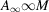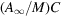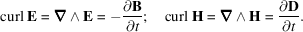International
Tables for
Crystallography
Volume D
Physical properties of crystals
Edited by A. Authier

International Tables for Crystallography (2006). Vol. D, ch. 1.1, pp. 11-12

## Section 1.1.4.3. Symmetries associated with an electric field and with magnetic induction (flux density)

A. Authiera*

aInstitut de Minéralogie et de la Physique des Milieux Condensés, Bâtiment 7, 140 rue de Lourmel, 75015 Paris, France
Correspondence e-mail: aauthier@wanadoo.fr

| top | pdf |

#### 1.1.4.3.1. Symmetry of an electric field

| top | pdf |

Considered as an effect, an electric field may have been produced by two circular coaxial electrodes, the first one carrying positive electric charges, the other one negative charges (Fig. 1.1.4.1). The cause possesses an axis of revolution and an infinity of mirrors parallel to it,. Considered as a cause, the electric field induces for instance the motion of a spherical electric charge parallel to itself. The associated symmetry is the same in each case, and the symmetry of the electric field is identical to that of a force,. The electric polarization or the electric displacement have the same symmetry.Figure 1.1.4.1 | top | pdf |Symmetry of an electric field.

#### 1.1.4.3.2. Symmetry of magnetic induction

| top | pdf |

The determination of the symmetry of magnetic quantities is more delicate. Considered as an effect, magnetic induction may be obtained by passing an electric current in a loop (Fig. 1.1.4.2). The corresponding symmetry is that of a cylinder rotating around its axis,. Conversely, the variation of the flux of magnetic induction through a loop induces an electric current in the loop. If the magnetic induction is considered as a cause, its effect has the same symmetry. The symmetry associated with the magnetic induction is therefore.Figure 1.1.4.2 | top | pdf |Symmetry of magnetic induction.

This symmetry is completely different from that of the electric field. This difference can be understood by reference to Maxwell's equations, which relate electric and magnetic quantities:It was seen in Section 1.1.3.8.3that the curl is an axial vector because it is a vector product. Maxwell's equations thus show that if the electric quantities (E, D) are polar vectors, the magnetic quantities (B, H) are axial vectors and vice versa; the equations of Maxwell are, in effect, perfectly symmetrical on this point. Indeed, one could have been tempted to determine the symmetry of the magnetic field by considering interactions between magnets, which would have led to the symmetryfor the magnetic quantities. However, in the world where we live and where the origin of magnetism is in the spin of the electron, the magnetic field is an axial vector of symmetrywhile the electric field is a polar vector of symmetry.